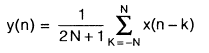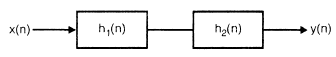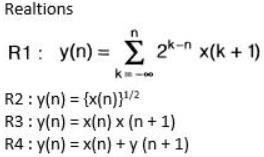Courses

# Signals & Systems - MCQ Test 1

## 10 Questions MCQ Test GATE Electrical Engineering (EE) 2022 Mock Test Series | Signals & Systems - MCQ Test 1

Description
This mock test of Signals & Systems - MCQ Test 1 for GATE helps you for every GATE entrance exam. This contains 10 Multiple Choice Questions for GATE Signals & Systems - MCQ Test 1 (mcq) to study with solutions a complete question bank. The solved questions answers in this Signals & Systems - MCQ Test 1 quiz give you a good mix of easy questions and tough questions. GATE students definitely take this Signals & Systems - MCQ Test 1 exercise for a better result in the exam. You can find other Signals & Systems - MCQ Test 1 extra questions, long questions & short questions for GATE on EduRev as well by searching above.
QUESTION: 1

Solution:
QUESTION: 2

Solution:
QUESTION: 3

### Let P be linearity, Q be time-invariance, R be the causality and S be the with memory. A continuous-time system has the input-output relationship: y(t) = t x(t) where y(t) is the output and x(t) is the input. The system has the properties.

Solution:
QUESTION: 4

The signal x(t) = t u(t) is a

Solution:
QUESTION: 5

The first derivatives of the signalx(t) = sgn t is

Solution:
QUESTION: 6

The non-invertible system is

Solution:
QUESTION: 7

The goup delay function  τ(ω) is related to phase function φ (ω) as

Solution:
QUESTION: 8

Let P be the linearity, Q be the Time-variant, R be the causality and S the static. A discrete­time system the input-output relationship,where the x(n) is an input and y(n) is an output. The above system has the properties

Solution:
QUESTION: 9

A disrete-time LTI susyem is described by,where, h1(n) = sin δn  h2(n) = an u(n); l a I < 1  and x(n) = 8(n) - a δ(n - 1)

Which one is the output y(n) ?

Solution:
QUESTION: 10

Match the system properties P1, P2 with the discrete time system relations R1, R2, R3 and R4.

Properties

P1 : Stable

P2 : CasualSolution: